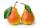# Pizza

Five friends were together for pizza. Adam divided his pizza into thirds, Boris in quarters, Denis in patina and Luke in sixth. Then Simon also came to them. Each of the five boys gave him one piece, leaving him one whole pizza. In how many equal parts did Ivan slice his pizza?

Correct result:

i =  20

#### Solution:

$1/3+1/4+1/5+1/6+1/i=1 \ \\ \ \\ i/3+i/4+i/5+i/6+1=i \ \\ \ \\ 3i=60 \ \\ \ \\ i=20$We would be very happy if you find an error in the example, spelling mistakes, or inaccuracies, and please send it to us. We thank you!Tips to related online calculators
Need help calculate sum, simplify or multiply fractions? Try our fraction calculator.
Do you have a linear equation or system of equations and looking for its solution? Or do you have quadratic equation?

## Next similar math problems:

• PearsThere were pears in the basket, I took two-fifths of them, and left six in the basket. How many pears did I take?
• Fraction + eqSolve following simple equation with fractions: -5/6(8+5b) = 75 + 5/3b
• OrchardThree-fifths of the orchard are apple trees, one-third of the trees are cherries. The remaining 5 trees are pears. How many trees are in the orchard?
• Unknown numberI think the number - its sixth is 3 smaller than its third.
• Cleaning windowsCleaning company has to wash all the windows of the school. The first day washes one-sixth of the windows of the school, the next day three more windows than the first day and the remaining 18 windows washes on the third day. Calculate how many windows ha
• One thirdIf 3/5 is 360, how much is 1/3?
• FractionsThree-quarters of an unknown number are 4/5. What is 5/6 of this unknown number?
• Simply equationSolve this equation for x: ?
• Equation with xSolve the following equation: 2x- (8x + 1) - (x + 2) / 5 = 9
• BicycleThe bicycle pedal gear has 36 teeth, the rear gear wheel has 10 teeth. How many times turns rear wheel, when pedal wheel turns 120x?
• Unknown numberIdentify unknown number which 1/5 is 40 greater than one tenth of that number.
• Simple equationSolve for x: 3(x + 2) = x - 18
• Equation with fractionsSolve equation: ? It is equation with fractions.
• Eq-fracSolve the following equation with fractions: h + 1/3 =5/3
• EquationSolve the equation: 1/2-2/8 = 1/10; Write the result as a decimal number.
• Simple equation 6Solve equation with one variable: X/2+X/3+X/4=X+4
• UN 1If we add to an unknown number his quarter, we get 210. Identify unknown number.